Geometry

# Area trapezoid

Area trapezoid, formulas and calculator for calculating area online. Formulas are given for all types of trapezoids and special cases for isosceles trapezoids.

Table with area trapezoid formulas (at the end of the page)

1

## Area trapezoid in height and two bases... preparation ...
a - base
b - base
h - height

2

## Area trapezoid in height and midline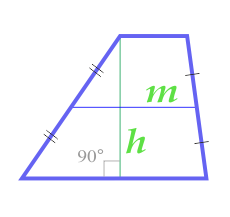... preparation ...
m - middle line
h - height

3

## Area trapezoid on four sides... preparation ...
a - base
b - base
c - side
d - side

4

## Area of trapezoid diagonally and the angle between the diagonals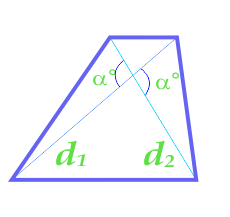... preparation ...
d1 - diagonal
d2 - diagonal
α° - Angle between diagonals

5

## Area of trapezoid through its base and the corners at the base... preparation ...
a - base
b - base
α° - angle at base
β° - angle at base

6

## Area of isosceles trapezoid through its sides... preparation ...
a - side
b - side
c - side

7

## Area of an isosceles trapezoid through a small base, lateral side and angle with a larger base... preparation ...
a - base
c - side
α° - angle at base

8

## Area of an isosceles trapezoid through a larger base, lateral side and angle with a larger base... preparation ...
b - base
c - side
α° - angle at base

9

## Area of isosceles trapezoid through the base and the angle at the base... preparation ...
a - base
b - base
α° - angle at base

10

## Area of isosceles trapezoid through the diagonals and the angle between the diagonals... preparation ...
d - diagonal
α° - Angle between diagonals

11

## Area of isosceles trapezoid through the midline, side and corner at the base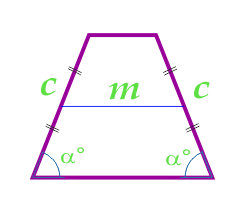... preparation ...
m - middle line
c - side
α° - angle between parties

12

## Area of isosceles trapezoid along the radius of the inscribed circle and the angle between the sidesThis formula is applicable only for isosceles trapezoids into which a circle can be entered.

... preparation ...
r - radius of inscribed circle
α° - angle between parties

13

## Area of isosceles trapezoid through its two bases and the radius of the inscribed circle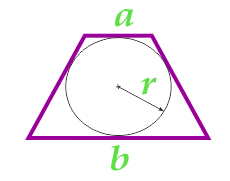This formula is applicable only for isosceles trapezoids into which a circle can be entered.

... preparation ...
a - base
b - base
r - radius of inscribed circle

14

## Area of isosceles trapezoid through its base and the angle with a larger baseThis formula is applicable only for isosceles trapezoids into which a circle can be entered.

... preparation ...
a - base
b - base
α° - angle at base

15

## Area of isosceles trapezoid across the sidesThis formula is applicable only for isosceles trapezoids into which a circle can be entered.

... preparation ...
a - base
b - base
c - side

16

## Area of isosceles trapezoid through the base and the midline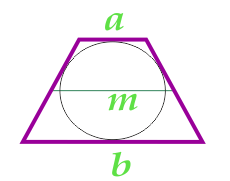This formula is applicable only for isosceles trapezoids into which a circle can be entered.

... preparation ...
a - base
b - base
m - middle line

Note:

If the angle is specified in radians in the source data, then for conversion to degrees you can use the formula: 1 radian × (180/π)° = 57,296°

## Table with area trapezoid formulas

Depending on the known initial data and the type of trapezoid, the area of trapezoid can be calculated using various formulas.

 initial data(active link to go to the calculator) sketch formula Area for all types of trapezoid 1 height and two bases 2 height and midline 3 four sides 4 diagonals and the angle between them 5 bases and angles at one of the bases Area of isosceles trapezoid 6 the parties 7 base, sides and corner at the base 8 base, sides and corner at the base 9 bases and angles at one of the bases 10 diagonals and the angle between them 11 midline, sides and angles between base and sides 12 radius of the inscribed circle and the angle at the base 13 base and radius of the inscribed circle 14 bases and angles at one of the bases 15 bases and sides 16 base and middle line